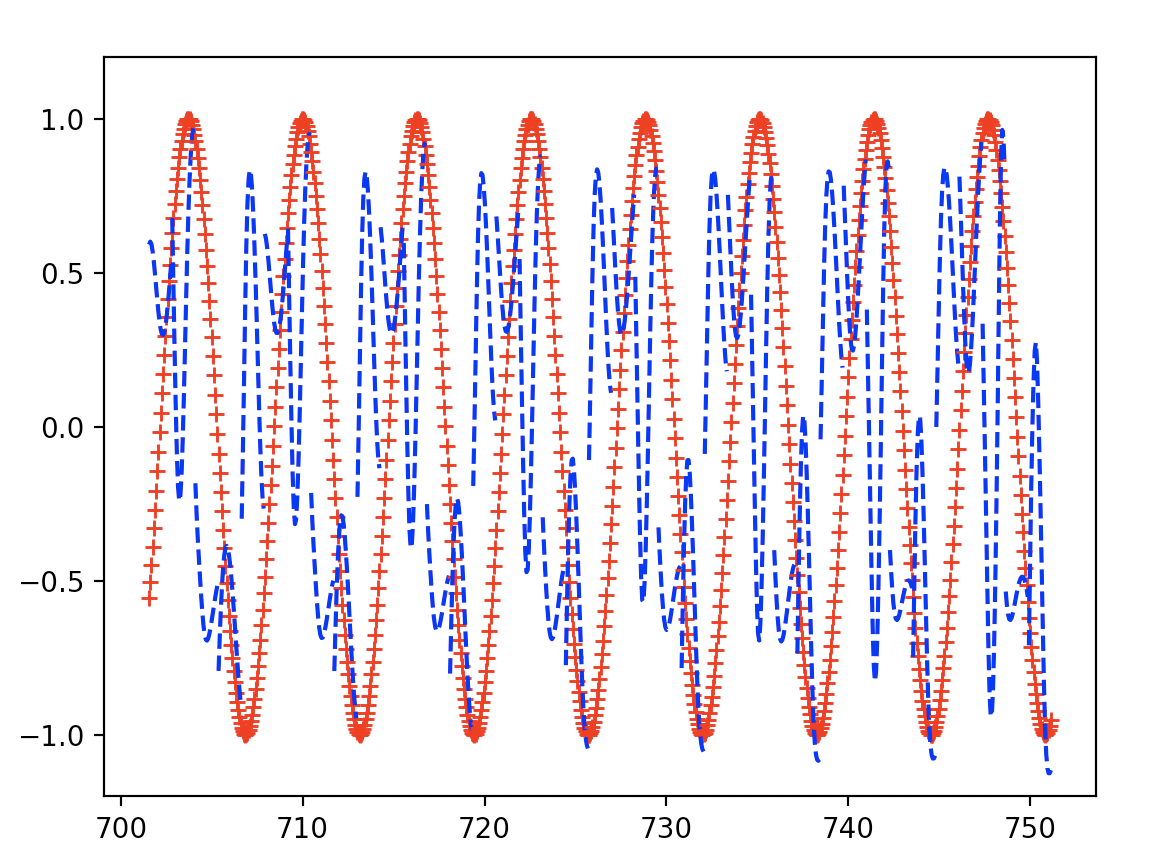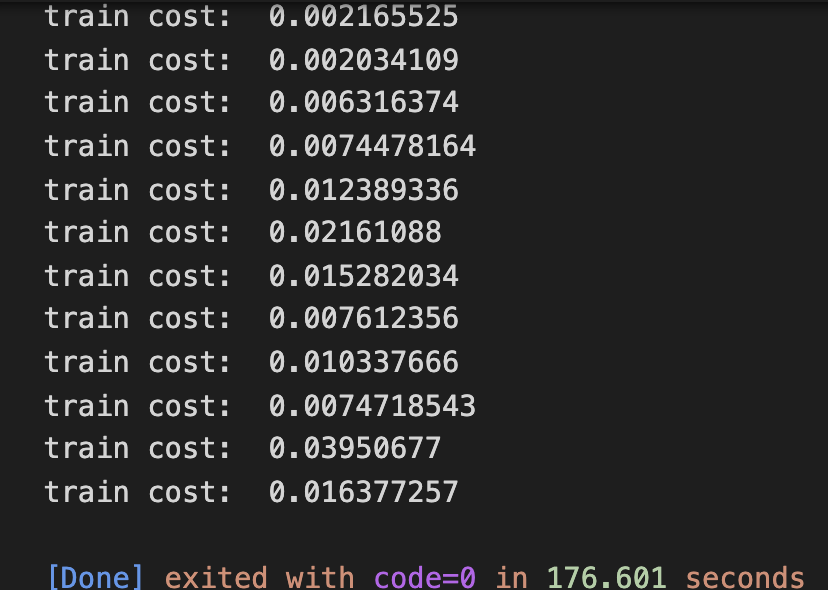• Hamilton（2018）提出了回归过滤器（Hamilton过滤器）作为Hodrick-Prescott过滤器（HP过滤器）的替代方案。 他认为汉密尔顿过滤器满足了HP过滤器用户的所有期望目标，同时解决了其缺点。 我表明，这两个目标之间存在...
• 这项工作的目的是在前馈人工神经网络（ANN）上应用和试验各种选择效果，该神经网络用于获得基于4个输入来预测联合循环电厂的电力输出功率（EP）的回归模型。 数据集是从开放的在线资源获得的。 这项工作显示并解释...
• RNN 循环神经网络 回归 地平线上的背影关注 RNN网络较少运用于回归任务，但是并不代表其不可运用于回归任务。本文通过简单回归任务的RNN进一步加深对RNN的理解 1. 准备数据和超参数 import torch from torch ...
RNN 循环神经网络 回归
地平线上的背影关注
RNN网络较少运用于回归任务，但是并不代表其不可运用于回归任务。本文通过简单回归任务的RNN进一步加深对RNN的理解
1. 准备数据和超参数
import torch
from torch import nn
import numpy as np
import matplotlib.pyplot as plt

# torch.manual_seed(1)    # reproducible

# Hyper Parameters
TIME_STEP = 10      # rnn time step
INPUT_SIZE = 1      # rnn input size
LR = 0.02           # learning rate

# show data
steps = np.linspace(0, np.pi*2, 100, dtype=np.float32)  # float32 for converting torch FloatTensor
x_np = np.sin(steps)
y_np = np.cos(steps)
plt.plot(steps, y_np, 'r-', label='target (cos)')
plt.plot(steps, x_np, 'b-', label='input (sin)')
plt.legend(loc='best')
plt.show()

2. 构建RNN神经网络
class RNN(nn.Module):
def __init__(self):
super(RNN, self).__init__()

self.rnn = nn.RNN(
input_size=INPUT_SIZE,
hidden_size=32,     # rnn hidden unit
num_layers=1,       # number of rnn layer
batch_first=True,
# input & output will has batch size as 1s dimension. e.g. (batch, time_step, input_size)
)
self.out = nn.Linear(32, 1)

def forward(self, x, h_state):
# x (batch, time_step, input_size)
# h_state (n_layers, batch, hidden_size)
# r_out (batch, time_step, hidden_size)
r_out, h_state = self.rnn(x, h_state)

outs = []    # save all predictions
for time_step in range(r_out.size(1)):    # calculate output for each time step
outs.append(self.out(r_out[:, time_step, :]))

# instead, for simplicity, you can replace above codes by follows
# r_out = r_out.view(-1, 32)
# outs = self.out(r_out)
# outs = outs.view(-1, TIME_STEP, 1)
# return outs, h_state

# or even simpler, since nn.Linear can accept inputs of any dimension
# and returns outputs with same dimension except for the last
# outs = self.out(r_out)
# return outs

rnn = RNN()
print(rnn)

3. 选择优化器和损失函数
optimizer = torch.optim.Adam(rnn.parameters(), lr=LR)   # optimize all cnn parameters
loss_func = nn.MSELoss()


注：

4. 初始化数据和plt图像
h_state = None      # for initial hidden state

plt.figure(1, figsize=(12, 5))
plt.ion()           # continuously plot

5. 训练和优化
for step in range(100):
start, end = step * np.pi, (step+1)*np.pi   # time range
# use sin predicts cos
steps = np.linspace(start, end, TIME_STEP, dtype=np.float32, endpoint=False)
# float32 for converting torch FloatTensor
x_np = np.sin(steps)
y_np = np.cos(steps)

x = torch.from_numpy(x_np[np.newaxis, :, np.newaxis])
# shape (batch, time_step, input_size)
y = torch.from_numpy(y_np[np.newaxis, :, np.newaxis])

prediction, h_state = rnn(x, h_state)   # rnn output
# !! next step is important !!
h_state = h_state.data
# repack the hidden state, break the connection from last iteration

loss = loss_func(prediction, y)         # calculate loss

# plotting
plt.plot(steps, y_np.flatten(), 'r-')
plt.plot(steps, prediction.data.numpy().flatten(), 'b-')
plt.draw(); plt.pause(0.05)

plt.ioff()
plt.show()
展开全文神经网络 python RNN
• ## foreach循环详细篇

千次阅读 2019-04-14 18:04:01
Java SE5引入了一种更加简洁的 for 语法用于数组 和 容器，即 ...使用 foreach 循环遍历数组和集合元素时，无须获得数组和集合长度，无须根据索引来访问数组元素和集合元素，foreach 循环自动遍历数组和集合的...

Java SE5引入了一种更加简洁的 for 语法用于数组 和 容器，即 foreach语法，表示不必创建int变量去对由访问项构成的序列进行计数，foreach将自动产生每一项。

foreach 循环，这种循环遍历数组 和 集合 更加简洁。 使用 foreach 循环遍历数组和集合元素时，无须获得数组和集合长度，无须根据索引来访问数组元素和集合元素，foreach 循环自动遍历数组和集合的每个元素。

foreach 循环的语法格式入下:
for (type variableName : array | collection)//array 数组 collection 容器/集合
{
// variableName 自动迭代访问每个元素...
}

type 是数组元素 或 集合元素的类型， variableName 是一个形参名，foreach 循环自动将数组元素、集合元素依次赋值给该变量。下列有个示例:
public static void main(String[] args){

String[ ] books = {"Hello","World","!"};
//使用foreach 循环来遍历数组元素
//其中 book 将会自动迭代每个数组元素
for(String book : books){
System.out.println(book);
}
}

foreach 循环和普通循环不同的是:，它无需循环条件，无需循环迭代语句，这些都由系统来完成，foreach 循环自动迭代数组的每个元素，当每个元素都被迭代一次后，foreach循环自动结束。
注意: 使用此类循环遍历时，通常不要对循环变量进行赋值。虽然这样语法是允许的，但是没有太大实际意义，而且极易容易引起错误。看如下程序:
public static void main(String[] args){

String[ ] books = {"Hello","World","!"};
//使用foreach 循环来遍历数组元素，
//其中 book 将会自动迭代每个数组元素。
for(String book : books){
book = "你好! 朋友";		 //注意此处
System.out.println(book);
}
System.out.println(books);//会发现并未改变数组元素的值
}

运行上面程序，结果如下:
	你好! 朋友
你好! 朋友
你好! 朋友
Hello

由于在 foreach 循环中对数组数组进行赋值，结果导致不正常遍历数组元素，不能正确的取出每个数组元素的值。而且当再次访问第一个数组元素时，发现数组元素的值依然没有改变。不难看出，当使用foreach来迭代访问数组元素时，foreach中的循环变量相当于一个临时变量，系统会把数组元素依次赋给这个临时变量，而这个临时变量并不是数组元素，它只是保存了数组元素的值。因此，如果希望改变数组元素的值，则不能使用这种 foreach 循环。
注意:使用 foreach 循环迭代数组元素时，并不能改变数组元素的值，因此不要对 foreach 的循环变量进行赋值。
展开全文• 当影响因变量的因素是多个时候，这种一个变量同时与多个变量的回归问题就是多元回归，分为：多元线性回归和多元非线性回归。这里直说多元线性回归。对比一元线性回归： 1.1多元回归模型： y=β0+β1x1+β2x2+…+β...
当影响因变量的因素是多个时候，这种一个变量同时与多个变量的回归问题就是多元回归，分为：多元线性回归和多元非线性回归。这里直说多元线性回归。对比一元线性回归：
1.1多元回归模型：

y=β0+β1x1+β2x2+…+βkxk+ε

y=\beta_{0}+\beta_{1}x_{1}+\beta_{2}x_{2}+\ldots+\beta_{k}x_{k}+\varepsilon

1.2多元回归方程

E(y)=β0+β1x1+β2x2+…+βkxk

E(y)=\beta_{0}+\beta_{1}x_{1}+\beta_{2}x_{2}+\ldots+\beta_{k}x_{k}

1.3估计的多元回归方程

y^=β0^+β1^x1+β2^x2+…+βk^xk

\hat{y}=\hat{\beta_{0}}+\hat{\beta_{1}}x_{1}+\hat{\beta_{2}}x_{2}+\ldots+\hat{\beta_{k}}x_{k}
2.1**对参数的最小二乘法估计：**
和一元线性回归中提到的最小二乘法估计一样、这不过这里的求导变量多了点、原理是一样的、这里需要借助计算机求导、就不写了。

3 回归方程的拟合优度：
3.1 多重判定系数：(Multiple coefficient of determination)

R^{2}=\frac{SSR}{SST}=1-\frac{SSE}{SST}

注解：

(1 ) 对于多重判定系数有一点特别重要的需要说明：自变量个数的增加将影响到因变量中被估计的回归方程所解释的变量数量。当增加自变量时，会使预测误差变得较小，从而减小残差平方和

SSE
$SSE$。自然就会是

SSR
$SSR$变大。自然就会是

R2
$R^{2}$变大。这就会引发一个问题。如果模型中增加一个自变量，即使这个自变量在统计上并不显著，

R2
$R^{2}$的值也会变大。因此为了避免这个问题。提出了

R2a=1−(1−R2)(n−1n−k−1)

R^{2}_{a}=1-(1-R^{2})(\frac{n-1}{n-k-1})

R2a
$R^{2}_{a}$ 同时考虑了样本量

(n)
$(n)$ 和模型中自变量的个数

(k)
$(k)$ 的影响，这就使得

R2a
$R^{2}_{a}$ 的值永远小于

R2
$R^{2}$，而且

R2a
$R^{2}_{a}$ 的值不会因为模型中自变量的个数增多而逐渐接近于

1
$1$.
(2 ) R2$R^{2}$ 的平方根成为多重相关系数，也称为复相关系数，
它度量了因变量同

k
$k$ 个自变量的相关程度。
3.2 估计标准误差
同一元线性回归一样，多元回归中的估计标准误差也是误差项 ε$\varepsilon$ 的方差

σ2
$\sigma^{2}$ 的一个估计值，

se=SSEn−k−1−−−−−−−−√=MSE−−−−−√

s_{e}=\sqrt{\frac{SSE}{n-k-1}}=\sqrt{MSE} 4. 显著性检验 在此重点说明，在一元线性回归中，线性关系的检验

(F检验)
$(F检验)$ 和回归系数的检验

(t检验)
$(t检验)$ 是等价的。 但是在多元回归中，线性关系的检验主要是检验因变量同多个自变量线性关系是否显著，在

k
$k$ 个自变量中，只要有一个自变量与因变量的线性关系显著， F检验$F检验$ 就能通过，但这不一定意味着每个自变量与因变量的关系都显著。回归系数检验则是对每个回归系数分别进行单独的检验，它主要用于检验每个自变量对因变量的影响是否都显著。如果某个自变量没有通过检验，就意味着这个自变量对因变量的影响不显著，也许就没有必要将这个自变量放进回归模型中。  4.1 线性关系的检验  步骤：  （1）：提出假设

H0:β1=β2=…=βk=0

H_{0}:\beta_{1}=\beta_{2}=\ldots=\beta_{k}=0

H1:β1,β2,…=βk至少有一个不等于0

H_{1}:\beta_{1},\beta_{2},\ldots=\beta_{k}至少有一个不等于0  （2）：计算检验的统计量F.

F=SSR/kSSE/(n−k−1)≈F(k,n−k−1)

F=\frac{SSR/k}{SSE/(n-k-1)}\approx F(k,n-k-1)  （3）：作出统计决策。  4.2 线性关系的检验  步骤：  （1）：提出假设

H0:βi=0

H_{0}:\beta_{i}=0

H1:βi≠0

H_{1}:\beta_{i}\neq0  （2）：计算检验的统计量F.

ti=βi^sβi^≈t(n−k−1)

t_{i}=\frac{\hat{\beta_{i}}}{s_{\hat{\beta_{i}}}}\approx t(n-k-1)  （3）：作出统计决策。 5.1 多重共线性 多重共线性：当回归模型中两个或两个以上的变量彼此相关时，则称回归模型中存在多重共线性。  多重共线性的判别：  （1）模型中中各对自变量之间显著相关  （2）当模型的线性关系检验

(F检验)
$(F 检验)$ 显著时，几乎所有的回归系数

βi
$\beta_{i}$ 的

t
$t$ 检验却不显著。
（3）回归系数的正负号与预期的相反。
（4）容忍度(tolerance) 与 方差扩大因子(variance inflation factor, VIF).
容忍度：某个变量的容忍度等于 1 减去该自变量为因变量而其他 k−1$k-1$ 个自变量为预测变量时所得到的线性回归模型的判定系数。即

1−R2i
$1-R^{2}_{i}$。 容忍度越小，多重共线性越严重。通常认为 容忍度小于 0.1 时，存在严重的多重共线性。  方差扩大因子：容忍度的倒数。 因此，

VIF
$VIF$ 越大，多重共线性越严重，一般认为

VIF
$VIF$ 的值大于10时，存在严重的多重共线性。 5.2 多重共线性的处理 常见的两种办法：  （1）将一个或多个相关的自变量从模型中剔除，使保留的自变量尽可能不相关。  （2）如果要在模型中保留所有的自变量，那么应该：  （2.1）避免根据

t
$t$统计量对单个参数 β$\beta$ 进行检验，  （2.2）对因变量

y
$y$ 值的推断（预测和估计）限定在自变量样本值的范围内。

5.3选择变量避免共线性的几种方式，

在建立回归模型时，我们总是希望用最少的变量来说明问题，选择自变量的原则通常是对统计量进行显著性检验，检验的根据是：将一个或一个以上的自变量引入回归模型中时，是否使残差平方和 (SSE)$(SSE)$ 显著减少，如果增加一个自变量使残差平方和

(SSE)
$(SSE)$ 显著减少，则说明有必要将这个变量引入回归模型中，否则，没有必要将这个变量引入回归模型中。确定在模型中引入自变量

xi
$x_{i}$ 是否使残差平方和

(SSE)
$(SSE)$ 显著减少的方法，就是使用

F
$F$ 统计量的值作为一个标准，以此来确定在模型中增加一个自变量，还是从模型中剔除一个自变量。
变量选择方式：
5.3.1 向前选择;
第一步： 对 k$k$ 个自变量分别与因变量

y
$y$ 的一元线性回归模型，共有 k$k$ 个，然后找到

F
$F$ 统计量的值最大的模型及其自变量 xi$x_{i}$ 并将其首先引入模型。  第二步： 在已经引入模型的

xi
$x_{i}$ 的基础上，再分别拟合

xi
$x_{i}$ 与模型外的

k−1
$k-1$ 个自变量的线性回归模型，挑选出

F
$F$ 值最大的含有两个自变量的模型， 依次循环、直到增加自变量不能导致 SSE$SSE$ 显著增加为止，  5.3.2向后剔除  第一步：先对所有的自变量进行线性回归模型。然后考察

p<k
$p 个去掉一个自变量的模型，使模型的SSE值减小最少的自变量被挑选出来从模型中剔除，  第二步：考察

p−1
$p-1$ 个再去掉一个自变量的模型，使模型的SSE值减小最少的自变量被挑选出来从模型中剔除，直到剔除一个自变量不会使SSE值显著减小为止，这时，模型中的所剩自变量自然都是显著的。  5.3.3逐步回归  是上面两个的结合、考虑的比较全，以后就用这个就可以。
具体的分析过程、咱们以spss的多元回归分析结果为例。
展开全文• 用Keras搭建RNN回归循环神经网络2.1.导入必要模块2.2.超参数设置2.3.构造数据2.4.搭建模型2.5.激活模型2.6.训练+测试 1.前言 这次我们用循环神经网络(RNN, Recurrent Neural Networks)进行回归（Regression），主要...


文章目录
1.前言2.用Keras搭建RNN回归循环神经网络2.1.导入必要模块2.2.超参数设置2.3.构造数据2.4.搭建模型2.5.激活模型2.6.训练+测试

1.前言
这次我们用循环神经网络(RNN, Recurrent Neural Networks)进行回归（Regression），主要用到LSTM RNN层。
2.用Keras搭建RNN回归循环神经网络
2.1.导入必要模块
import numpy as np
import matplotlib.pyplot as plt
from keras.models import Sequential
from keras.layers import LSTM, TimeDistributed, Dense
np.random.seed(42)

2.2.超参数设置
BATCH_START = 0
TIME_STEPS = 20    #序列步长为20
BATCH_SIZE = 50    #每次训练的BATCH_SIZE为50
INPUT_SIZE = 1     #输入维度
OUTPUT_SIZE = 1    #输出维度
CELL_SIZE = 20     #LSTM输出维度
LR = 0.001         #学习率

2.3.构造数据
我们使用RNN来求解回归(Regression)问题. 首先生成序列sin(x),对应输出数据为cos(x),设置序列步长为20，每次训练的BATCH_SIZE为50
def get_batch():
global BATCH_START, TIME_STEPS
xs = np.arange(BATCH_START,BATCH_START+TIME_STEPS*BATCH_SIZE).reshape((BATCH_SIZE,TIME_STEPS)) / (10*np.pi)
seq = np.sin(xs)
res = np.cos(xs)
BATCH_START += TIME_STEPS
#plt.plot(xs[0, :], res[0, :], 'r', xs[0, :], seq[0, :], 'b--')
#plt.show()
return [seq[:,:,np.newaxis],res[:,:,np.newaxis],xs]

2.4.搭建模型
然后添加LSTM RNN层，输入为训练数据，输出数据大小由CELL_SIZE定义。因为每一个输入都对应一个输出，所以return_sequences=True。 每一个点的当前输出都受前面所有输出的影响，BATCH之间的参数也需要记忆，故stateful=True
最后添加输出层，LSTM层的每一步都有输出，使用TimeDistributed函数。
model = Sequential()    #用于一层一层搭建网络
batch_input_shape = (BATCH_SIZE,TIME_STEPS,INPUT_SIZE),
output_dim = CELL_SIZE,     #输出数据大小
return_sequences = True,    #每一个输入都对应一个输出，所以return_sequences=True
stateful = True             #每一个点的当前输出都受前面所有输出的影响，BATCH之间的参数也需要记忆，故stateful=True
))

2.5.激活模型
model.compile(optimizer=adam,
loss = 'mse')

2.6.训练+测试
print('Training...............')
for step in range(1000):
X_batch, Y_batch, xs = get_batch()
cost = model.train_on_batch(X_batch,Y_batch)
pred = model.predict(X_batch,BATCH_SIZE)
plt.plot(xs[0,:],Y_batch.flatten(),'r+',xs[0,:],pred.flatten()[:TIME_STEPS],'b--')
plt.ylim(-1.2,1.2)
plt.draw()
plt.pause(0.1)
if step%50==0:
print('train cost:',cost)

设置优化方法，loss函数和metrics方法之后就可以开始训练了。 训练1000次，调用matplotlib函数采用动画的方式输出结果。
拟合图：打印loss：展开全文Keras 数据挖掘 Python 神经网络
• 1.1 回归： Investpedia: Regression refers to the relation between selected values of x and observed values of y (from which the most probable value of y can be predicted for any value of x). ...
• 主要介绍了JavaScript求一组数的最小公倍数和最大公约数常用算法,结合实例形式分析了javascript基于面向对象，回归迭代和循环等算法求解一组数的最小公倍数与最大公约数相关操作技巧,需要的朋友可以参考下
• 数据挖掘线性回归数据集，用于数据挖掘课本中第六章回归案例实验使用。数据挖掘线性回归数据集，用于数据挖掘课本中第六章回归案例实验使用。
• 在这些示例中，使用循环和随机坐标下降算法解决了具有L1正则化的LASSO问题。 该算法的一般形式为： 例子： 此存储库中有三个示例： 使用真实数据集的演示 使用模拟数据集的演示 我的算法与Sklearn的比较 数据集：...Python
• ## 线性回归-理论篇

千次阅读 2014-07-03 12:11:38
线性回归（Linear Regression） "回归"的由来 Francis Galton,英国生物学家，他研究了父母身高与子女身高之间关系后得出，若父母身高高于平均大众身高，则其子女身高倾向于倒退生长，即会比其父母身高矮...机器学习 梯度下降
• 从本专栏开始，作者正式开始研究Python深度...本篇文章将分享循环神经网络LSTM RNN如何实现回归预测，通过sin曲线拟合实现如下图所示效果。本文代码量比较长，但大家还是可以学习下的。基础性文章，希望对您有所帮助！人工智能 LSTM RNN TensorFlow
• bpnreg的目标是为循环数据拟合贝叶斯投影法线回归模型。 安装 可以从CRAN安装R软件包bpnreg，如下所示： install.packages( " bpnreg " ) 您可以使用以下命令从github安装bpnreg的beta版本： # install.packages...R
• 以不可知的方式对多元时间序列进行建模，不假设潜在的理论结构，传统上是使用矢量自动回归进行的。 它们非常适合线性和与状态无关的进化。 多元循环神经网络的更通用方法允许捕获非线性和状态相关的动态。 本文采用...
• 1.单变量线性回归> y(5,7,9,11,16,20) > x(1,2,3,4,7,9) > lsfit(x,y) $coefficients Intercept X 3.338028 1.845070$residuals  -0.18309859 -0.02816901 0.12676056 0.28169014 -0.25352113 0.056r语言
• #4.3_RNN_循环神经网络_回归_(PyTorch_tutorial_神经网络_教学)学习资源
• 主要介绍了Python利用神经网络解决非线性回归问题,结合实例形式详细分析了Python使用神经网络解决非线性回归问题的相关原理与实现技巧,需要的朋友可以参考下
• ML之SLR：简单线性回归；根据多组数据(x,y)模拟得到一次线性方程(斜率和截距)，然后输入新的x来智能预测y值 目录 输出结果 代码设计 输出结果 代码设计 import numpy as np def fitSLR(x...
• @(Aaron)[机器学习 | 循环神经网络] 主要内容包括： 基于循环神经网络的语言模型，提供了从零开始的实现与简洁实现 代码实践 文章目录循环神经网络的构造裁剪梯度代码实践 循环神经网络的构造   循环神经网络...神经网络 机器学习 人工智能 python
• 这次我们会使用RNN来进行回归（Regression）的训练，使用自己创建的sin曲线预测一条cos曲线。如下图所示，我们用蓝色的sin曲线预测红色的cos曲线。 二、回归预测要实现的效果 经过RNN的回归训练，我们的网络预测...Tensorflow RNN LSTM BPTT
• 因此，就有了现在的循环神经网络，他的本质是：拥有记忆的能力，并且会根据这些记忆的内容来进行推断。因此，他的输出就依赖于当前的输入和记忆。 网络结构及原理 循环神经网络的基本结构特别简单，就是将网络的输出...
• #使用RNN进行回归训练，会用到自己创建对sin曲线，预测一条cos曲线， #【1】设置RNN各种参数 #import state as state import tensorflow as tf import numpy as np import matplotlib.pyplot as plt ...RNN
• 一、if语句 注意： a b if(a>b) { print(“a wins!”) } else if(ar语言 应用
• 循环神经网络让神经网络有了记忆, 对于序列话的数据,循环神经网络能达到更好的效果. 如果你对循环神经网络还没有特别了解, 请观看几分钟的短动画, RNN 动画简介 和 LSTM 动画简介 能让你生...RNN pytorch
• 循环神经网络（Recurrent Neural Network, RNN）是一类以序列（sequence）数据为输入，在序列的演进方向进行递归（recursion）且所有节点（循环单元）按链式连接的递归神经网络（recursive neural network） 对循环...
• 神经网络模型一般用来做分类，回归预测模型不常见，本文基于一个用来分类的BP神经网络，对它进行修改，实现了一个回归模型，用来做室内定位。模型主要变化是去掉了第三层的非线性转换，或者说把非线性激活函数...
• 回归模型目标函数 一、岭回归模型 1、介绍 Ridge/RidgeCV：使用结构风险最小化=损失函数（平方损失）+正则化（L2范数） Ridge 回归通过对系数的大小施加惩罚来解决 普通最小二乘法 的一些问题。 岭系数最小化的是...
• 一种新型带门限回归单元循环神经网络的信息安全评估算法.pdf
• 如此循环，直到系数收敛或达到指定的循环控制阈值。多分类的逻辑回归问题可转换为二分类来解决，因此需要为每一个分类在训练数据表中设定结果值列，结合训练样本列簇，按块批量梯度下降算法得到每个分类的回归系数。...多分类 大数据 HBase
• 一元回归分析和多元线性回归 前言 在统计学中，回归分析(Regression Analysis)指的是确定两种或两种以上变量间的相互依赖的定量关系的一种分析方法。该方法常使用数据统计的基本原理，对大量统计数据进行数学处理......